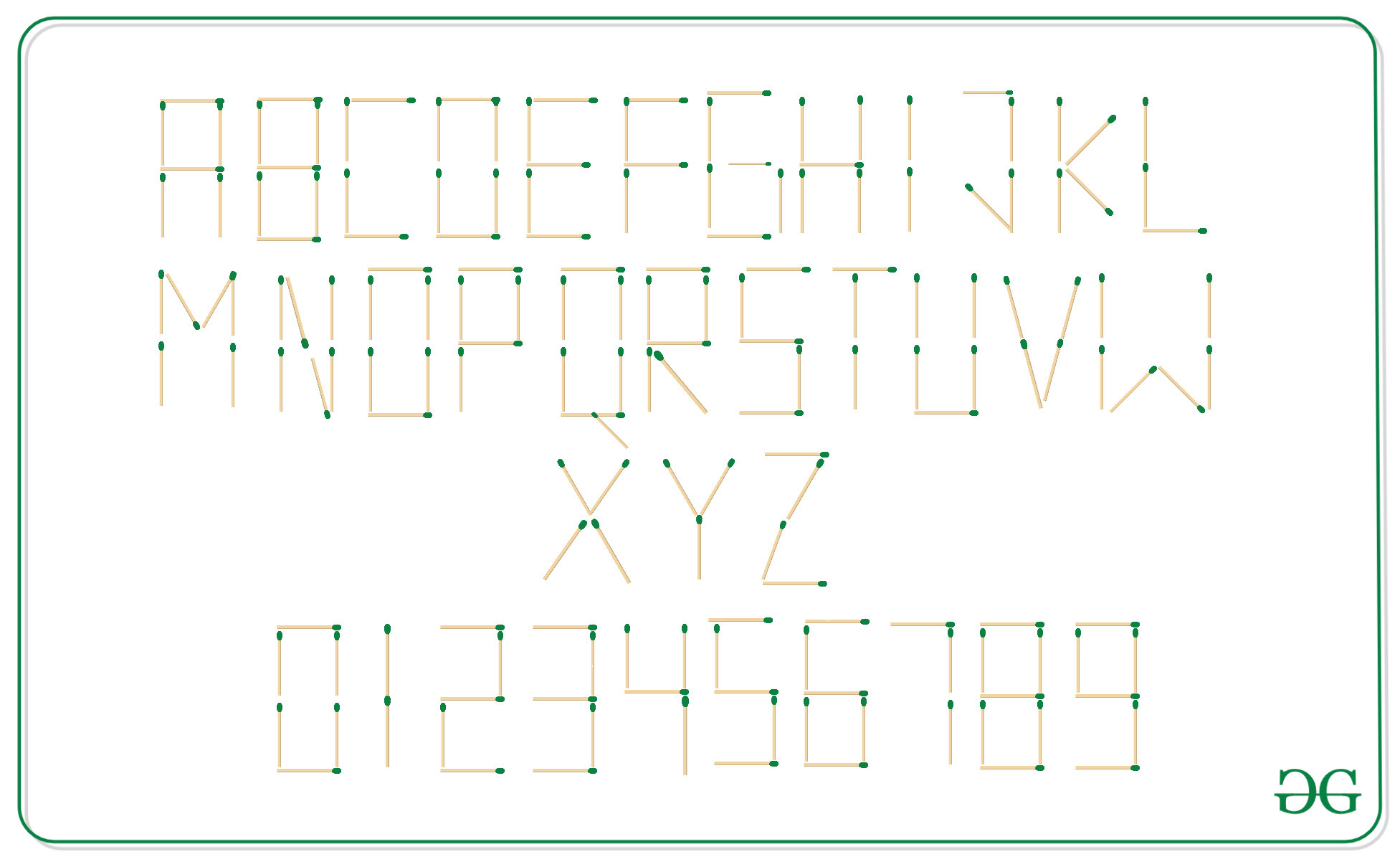# Sort the strings based on the numbers of matchsticks required to represent them

• Difficulty Level : Basic
• Last Updated : 17 Nov, 2021

Given an array arr[] of N strings, the task is to sort these strings according to the numbers of sticks required to represent them.

Examples:

Attention reader! Don’t stop learning now. Get hold of all the important DSA concepts with the DSA Self Paced Course at a student-friendly price and become industry ready.  To complete your preparation from learning a language to DS Algo and many more,  please refer Complete Interview Preparation Course.

In case you wish to attend live classes with experts, please refer DSA Live Classes for Working Professionals and Competitive Programming Live for Students.

Input: arr[] = { “123”, “ABC”, “88” }
Output: 123 88 ABC
Explanation:
Sticks required by each string are as follows:
123 -> 12 sticks
88 -> 14 sticks
ABC -> 17 sticks

Input: arr[] = { “GEEKS”, “FOR”, “GEEKSFORGEEKS” }
Output: FOR GEEKS GEEKSFORGEEKS
Explanation:
Sticks required by each string are as follows:
FOR => 16 sticks
GEEKS => 25 sticks
GEEKSFORGEEKS => 66 sticks

Approach: The idea is to compute the number of sticks required by each string with the help of the counts of the sticks required for each character. Then, store the count of the sticks and the strings into an array of pairs. Finally, sort them according to the number of sticks required.
The figure below is shown with the count of sticks required to represent each character:Below is the implementation of the above approach:

## C++

 `// C++ implementation to sort the``// strings with the number of``// sticks required to represent them` `#include ` `using` `namespace` `std;` `// Stick[] stores the count``// of sticks required to``// represent the alphabets``int` `sticks[] = { 6, 7, 4, 6, 5, 4, 6,``                 ``5, 2, 4, 4, 3, 6, 6,``                 ``6, 5, 7, 6, 5, 3, 5,``                 ``4, 6, 4, 3, 4 };` `// Number[] stores the count``// of sticks required to``// represent the numerals``int` `number[] = { 6, 2, 5, 5, 4, 5, 6,``                 ``3, 7, 6 };` `// Function that return the count of``// sticks required to represent``// the given string str``int` `countSticks(string str)``{``    ``int` `cnt = 0;` `    ``// Loop to iterate over every``    ``// character of the string``    ``for` `(``int` `i = 0; str[i]; i++) {` `        ``char` `ch = str[i];` `        ``// Add the count of sticks``        ``// required to represent the``        ``// current character``        ``if` `(ch >= ``'A'` `&& ch <= ``'Z'``) {``            ``cnt += sticks[ch - ``'A'``];``        ``}``        ``else` `{``            ``cnt += number[ch - ``'0'``];``        ``}``    ``}``    ``return` `cnt;``}``// Function to sort the array``// according to the number of``// sticks required to represent it``void` `sortArr(string arr[], ``int` `n)``{``    ``// Vector to store the number``    ``// of sticks required with``    ``// respective strings``    ``vector > vp;` `    ``// Inserting number of sticks``    ``// with respective strings``    ``for` `(``int` `i = 0; i < n; i++) {``        ``vp.push_back(``            ``make_pair(countSticks(arr[i]),``                      ``arr[i]));``    ``}` `    ``// Sort the vector,``    ``sort(vp.begin(), vp.end());` `    ``// Print the sorted vector``    ``for` `(``int` `i = 0; i < vp.size(); i++)``        ``cout << vp[i].second << ``" "``;``}` `// Driver Code``int` `main()``{``    ``string arr[] = { ``"GEEKS"``, ``"FOR"``,``                     ``"GEEKSFORGEEKS"` `};``    ``int` `n = ``sizeof``(arr) / ``sizeof``(arr);` `    ``sortArr(arr, n);` `    ``return` `0;``}`

## Java

 `// Java implementation to sort the``// strings with the number of``// sticks required to represent them` `import` `java.io.*;``import` `java.util.*;` `class` `GFG{``    ``// Stick[] stores the count``    ``// of sticks required to``    ``// represent the alphabets``    ``static` `int` `sticks[] = { ``6``, ``7``, ``4``, ``6``, ``5``, ``4``, ``6``,``                    ``5``, ``2``, ``4``, ``4``, ``3``, ``6``, ``6``,``                    ``6``, ``5``, ``7``, ``6``, ``5``, ``3``, ``5``,``                    ``4``, ``6``, ``4``, ``3``, ``4` `};``    ` `    ``// Number[] stores the count``    ``// of sticks required to``    ``// represent the numerals``    ``static` `int` `number[] = { ``6``, ``2``, ``5``, ``5``, ``4``, ``5``, ``6``,``                    ``3``, ``7``, ``6` `};``    ` `    ``// Function that return the count of``    ``// sticks required to represent``    ``// the given string str``    ` `    ``static` `class` `Pair ``implements` `Comparable{``        ``int` `num_sticks;``        ``String str;``        ` `        ``Pair(``int` `n, String s)``        ``{``            ``num_sticks = n;``            ``str=s;``        ``}``        ` `        ``public` `int` `compareTo(Pair p)``        ``{``            ``return` `this``.num_sticks-p.num_sticks;``        ``}``    ``}``    ``static` `int` `countSticks(String str)``    ``{``        ``int` `cnt = ``0``;``        ``int` `n=str.length();``        ` `        ``// Loop to iterate over every``        ``// character of the string``        ``for` `(``int` `i = ``0``; i < n; i++) {``    ` `            ``char` `ch = str.charAt(i);``    ` `            ``// Add the count of sticks``            ``// required to represent the``            ``// current character``            ``if` `(ch >= ``'A'` `&& ch <= ``'Z'``) {``                ``cnt += sticks[ch - ``'A'``];``            ``}``            ``else` `{``                ``cnt += number[ch - ``'0'``];``            ``}``        ``}``        ``return` `cnt;``    ``}``    ` `    ``// Function to sort the array``    ``// according to the number of``    ``// sticks required to represent it``    ``static` `void` `sortArr(String arr[], ``int` `n) ``    ``{``        ``// ArrayList to store the number``        ``// of sticks required with``        ``// respective strings``        ``ArrayList list = ``new` `ArrayList<>();``    ` `        ``// Inserting number of sticks``        ``// with respective strings``        ``for` `(``int` `i = ``0``; i < n; i++) {``            ``list.add(``                ``new` `Pair(countSticks(arr[i]),``                        ``arr[i]));``        ``}``    ` `        ``// Sort the list,``        ``Collections.sort(list);``    ` `        ``// Print the sorted vector``        ``for` `(``int` `i = ``0``; i < list.size(); i++)``            ``System.out.print(list.get(i).str + ``" "``);``    ``}``    ` `    ``// Driver Code``    ``public` `static` `void` `main(String []args)``    ``{``        ``String arr[] = { ``"GEEKS"``, ``"FOR"``,``                        ``"GEEKSFORGEEKS"` `};``        ``int` `n = arr.length;``    ` `        ``sortArr(arr, n);``    ``}``}`

## Python3

 `# Python3 implementation to sort the``# strings with the number of``# sticks required to represent them` `# Stick[] stores the count``# of sticks required to``# represent the alphabets``sticks ``=` `[``6``, ``7``, ``4``, ``6``, ``5``, ``4``, ``6``,``          ``5``, ``2``, ``4``, ``4``, ``3``, ``6``, ``6``,``          ``6``, ``5``, ``7``, ``6``, ``5``, ``3``, ``5``,``          ``4``, ``6``, ``4``, ``3``, ``4``]` `# Number[] stores the count``# of sticks required to``# represent the numerals``number ``=` `[``6``, ``2``, ``5``, ``5``, ``4``,``          ``5``, ``6``, ``3``, ``7``, ``6``]` `# Function that return the count of``# sticks required to represent``# the given string str``def` `countSticks(st):` `    ``cnt ``=` `0` `    ``# Loop to iterate over every``    ``# character of the string``    ``for` `i ``in` `range``(``len``(st)):` `        ``ch ``=` `st[i]` `        ``# Add the count of sticks``        ``# required to represent the``        ``# current character``        ``if` `(ch >``=` `'A'` `and` `ch <``=` `'Z'``):``            ``cnt ``+``=` `sticks[``ord``(ch) ``-``                          ``ord``(``'A'``)]       ``        ``else` `:``            ``cnt ``+``=` `number[``ord``(ch ) ``-``                          ``ord``(``'0'``)]``       ` `    ``return` `cnt` `# Function to sort the array``# according to the number of``# sticks required to represent it``def` `sortArr(arr, n):` `    ``# Vector to store the number``    ``# of sticks required with``    ``# respective strings``    ``vp ``=` `[]` `    ``# Inserting number of sticks``    ``# with respective strings``    ``for` `i ``in` `range``(n):``        ``vp.append([countSticks(arr[i]),``                               ``arr[i]])``   ` `    ``# Sort the vector``    ``vp.sort()` `    ``# Print the sorted vector``    ``for` `i ``in` `range``(``len``(vp)):``        ``print` `(vp[i][``1``], end ``=` `" "``)` `# Driver Code``if` `__name__ ``=``=` `"__main__"``:` `    ``arr ``=` `[``"GEEKS"``, ``"FOR"``,``           ``"GEEKSFORGEEKS"``]``    ``n ``=` `len``(arr)``    ``sortArr(arr, n)` ` ``# This code is contributed by Chitranayal`

## Javascript

 ``
Output:
`FOR GEEKS GEEKSFORGEEKS`

Time Complexity: O(N * log N)

Auxiliary space: O(N)

My Personal Notes arrow_drop_up